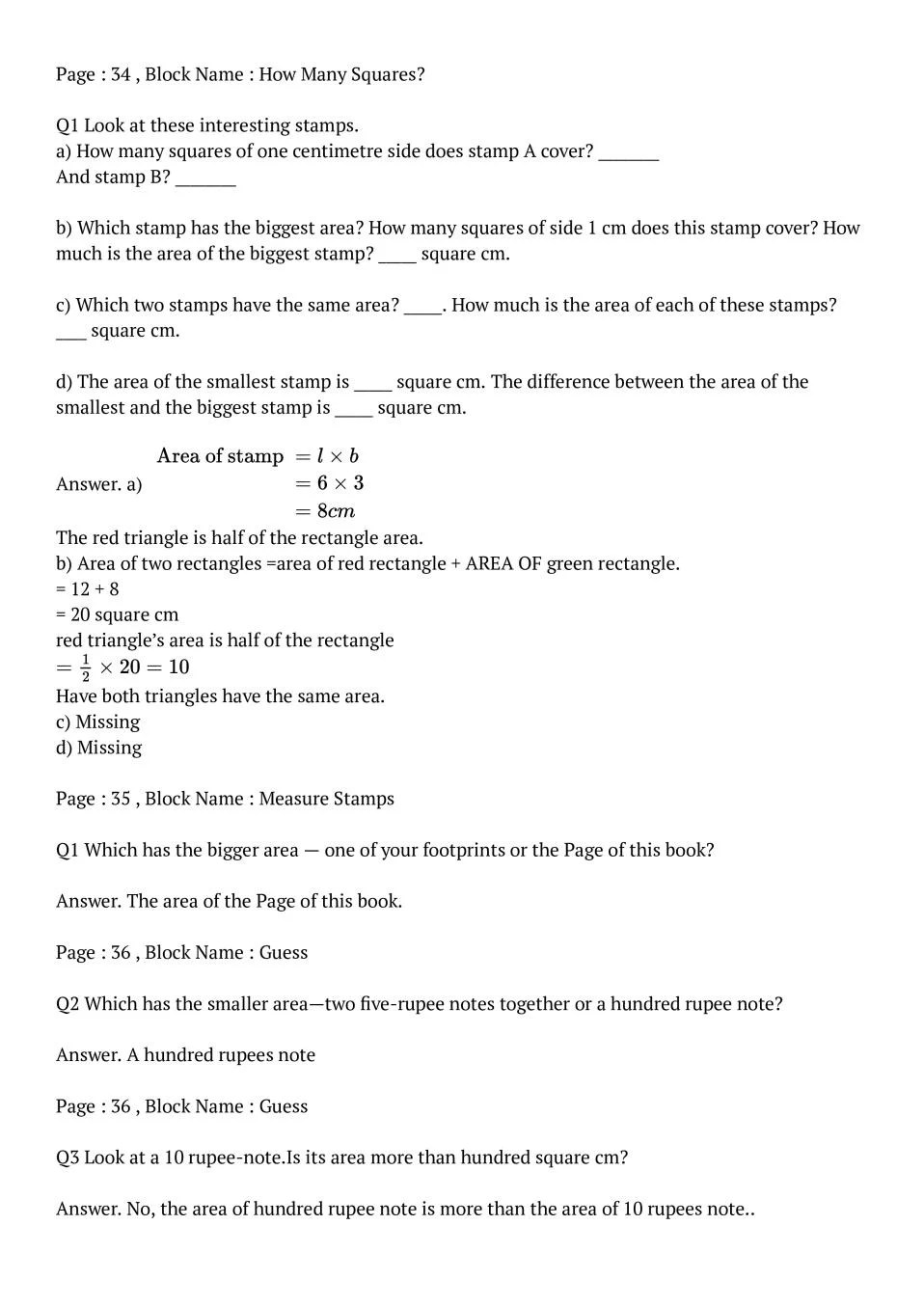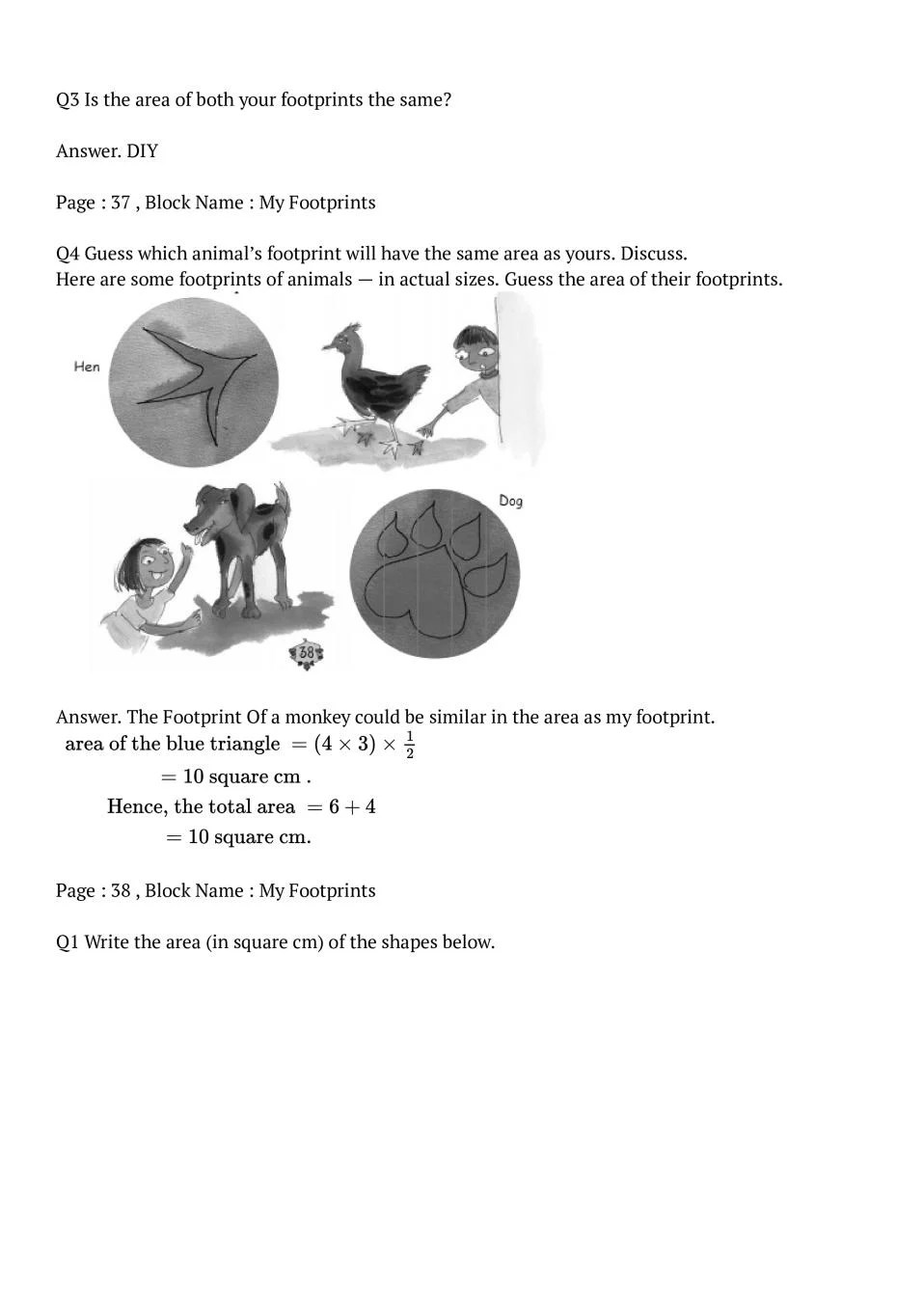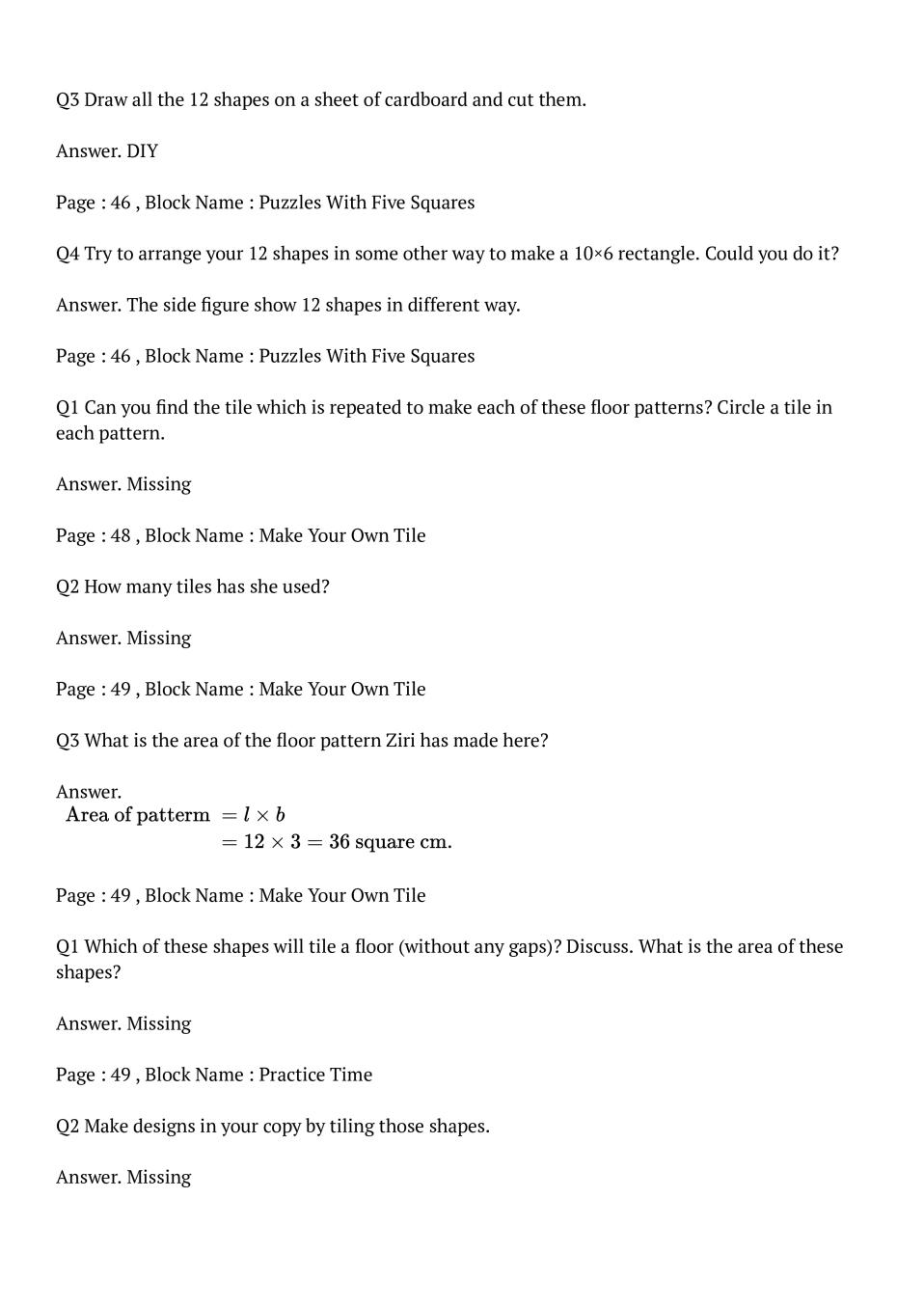# NCERT Solutions Class 5 Maths Chapter 3 How Many Squares?

Here you can get free NCERT Solutions for Class 5 Maths, of Chapter 3 How Many Squares? All NCERT Book Solutions are given here exercise wise for How Many Squares? NCERT Solutions are helpful in the preparation of several school level, graduate and undergraduate level competitive exams. Practicing questions from NCERT Maths Book, its Solutions for Class 5 Chapter 3 How Many Squares? is proven to enhance your subject skills.

 Class: 5th Class Chapter: Chapter 3 Name: How Many Squares? Subject: Maths

## NCERT Solutions Class 5 Maths Chapter 3 – How Many Squares?

NCERT Class 5 Solutions Chapter 3 – How Many Squares? are given below.

NCERT Solutions for Class 5 Maths (Math Magic) Chapter 3 How Many Squares?### Class 5 Maths Chapter 3 NCERT Solutions

Get step by step solutions for the questions given in class 5 Maths textbook from the latest NCERT as per board exam guidelines. We also suggest you to practice all the solved examples given in the NCERT book to clear your concepts on How Many Squares? You can buy NCERT Books for Class 5 Maths online for doorstep delivery.

### NCERT Solutions Class 5 Chapter Wise

If you want NCERT solutions of any topic other than Chapter 3 How Many Squares? check it from here. Maths Book by NCERT has all chapters that are in your Class 5 syllabus.

### NCERT Solutions Class 5 All Subjects

All NCERT Solutions for Subjects other than English are provided below:

 English Hindi Maths EVS

### Other Classes NCERT Solutions

If you want NCERT solutions of any class other than Class 5, check it from here.